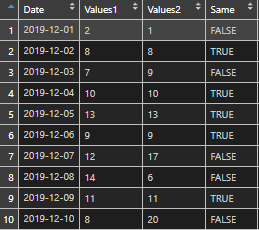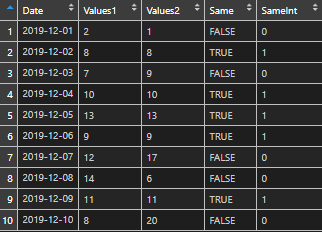# Convert R TRUE and FALSE values to 1 and 0, and vice versa

Here is a simple method to convert R TRUE and FALSE values to 1 and 0, and vice versa.

Here is my data frame.

```df <- data.frame(
Date  = as.POSIXct(c("2019-12-01", "2019-12-02", "2019-12-03", "2019-12-04", "2019-12-05", "2019-12-06", "2019-12-07", "2019-12-08", "2019-12-09", "2019-12-10")),
Values1 = as.numeric(c("2", "8", "7", "10", "13", "9", "12", "14", "11", "8")),
Values2 = as.numeric(c("1", "8", "9", "10", "13", "9", "17", "6", "11", "20")))```

If I’m doing some calculation that gives me TRUE or FALSE then sometimes it is necessary to convert them to 1 and 0.

`df\$Same <- df\$Values1 == df\$Values2`The easiest way is by using a function as.integer.

`df\$SameInt <- as.integer(df\$Same)`## Convert R 1 and 0 values to TRUE and FALSE

Sometimes it’s another way around. Here you go.

`df\$SameLogic <- as.logical(df\$SameInt)`

If you want to know how to reflow your code or other useful RStudio tips and tricks, take a look at this post.

Posted

in